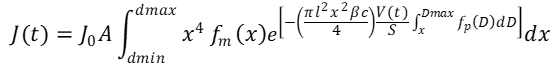cancel
Showing results for
Did you mean:
cancel
Showing results for
Did you mean:

Highlighted

## Equation solving challenge-Filtration

Hi everyone, for a second time I’ll ask for your help, I still working on a particle retention research and I would be really thankful if someone could help me out with this.

Let me explain the situation: I need to solve an equation that allows me to match experimental data to the Filippov model and here begins the problems, the experimental data represents a discrete distribution for particle and pore size distribution, but the Filippov model used a continuous log-normal distribution function for particles and bilog-normal distribution function for membrane pores, I’ve been trying to use the “dlnorm” function from Mathcad but I suppose that  something is wrong because it doesn’t work like it supposed to do. I’ve been reading a paper that claims to solve the integro-diffrential equations for flux J(t) and for the injected volume V(t), this paper however, does not give much detail about it.

Thank you in advance, I am open to advices related to this particular challenge.

4 REPLIES 4

## Re: Equation solving challenge-Filtration

You have a complex problem!

You're right, the article is hard to read and doesn't give much detail.

Unfortunately your worksheet is hard (for me) to understand too.  It's not exactly clear what you're trying to do.  Some of your sheet has units, some does not, gets very challenging to track and understand.

Maybe a little more description of what you're trying to do?

## Re: Equation solving challenge-Filtration

Thanks for your answer, maybe this worksheet could help. Like I said, I'm trying to plot J(t) and V(t) that it is the solution of the IDE (integro-differential equation).

Regards

## Re: Equation solving challenge-Filtration

Okay.  Look at the attached sheet.

I've started putting units to some of your quantities (not just typing them beside unitless values.

Then I got as far as needing to define flux  J(t) as a function so it could be evaluated.  Can't find (in the article) how to do that so I'm stumped unless you can shed some light.

## Re: Equation solving challenge-Filtration

Thanks for your answer, I simplified a lot of things on the worksheet . Like I said, I'm trying to plot J(t) and V(t) that it is the solution of the IDE (integro-differential equation). The part that is confusing, it is that probability used J(t) to be calculated, as you can see in the equation, this is one of the equation that I want to plot (the probability was already substituted assuming continuous distrution)Regards